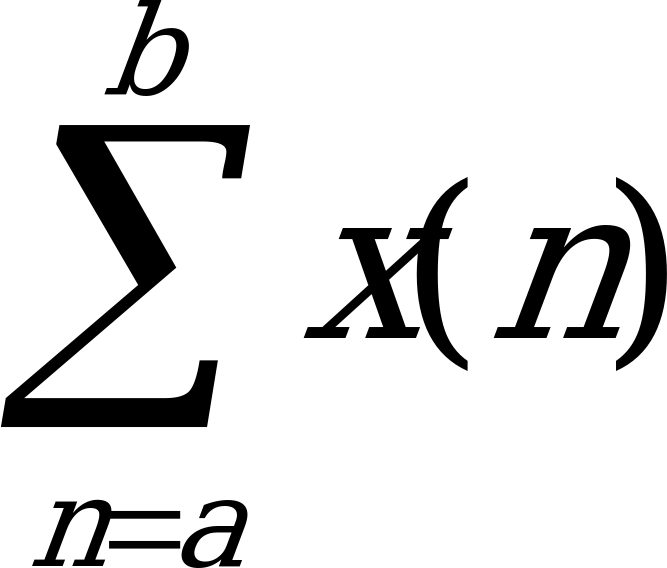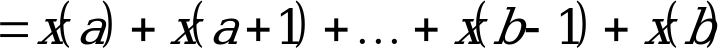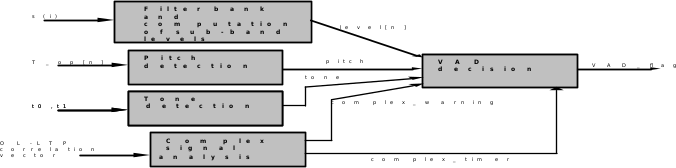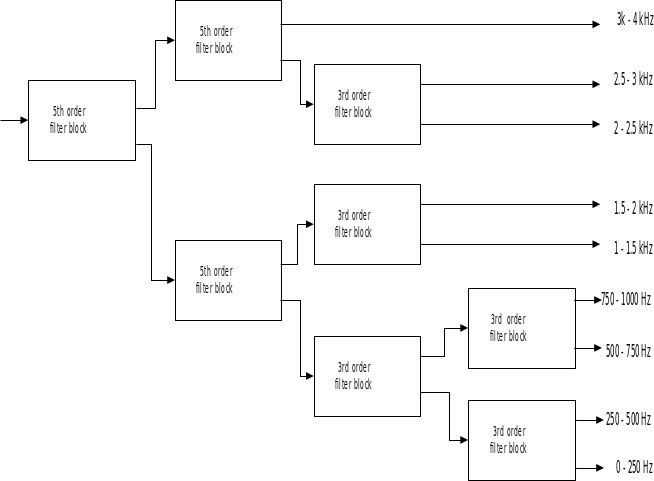## 3 Technical Description of VAD Option 1

06.943GPPTSVoice Activity Detector (VAD) for Adaptive Multi Rate (AMR) speech traffic channels

## 3.1 Definitions, symbols and abbreviations

### 3.1.1 Definitions

For the purposes of the presenty document, the following terms and definitions apply:

frame: Time interval of 20 ms corresponding to the time segmentation of the speech transcoder.

### 3.1.2 Symbols

For the purposes of the present document, the following symbols apply.

#### 3.1.2.1 Variables

bckr_est[n] background noise estimate

burst_count counts length of a speech burst, used by VAD hangover addition

complex_hang_timer hangover initator, used fo Complex Activity Estimation

lagcount pitch detection counter

level[n] signal level

new_speech pointer of the speech encoder, points a buffer containing last received samples of a speech frame 

noise_level average level of the background noise estimate

oldlagcount lagcount of the previous frame

pitch flag indicating presence of a periodic signal

complex_warning flag indicating the presence of a complex signal.

best_corr_hp normalized and limited value from maximum HP filtered correlation vector

corr_hp filtered best_corr_hp values

pow_sum power of the input frame

s(i) samples of the input framer

snr_sum measure between input frame and noise estimate

stat_count stationarity counter

stat_rat measure indicating stationary

T_op[n] open-loop lags 

t0 autocorrelation maxima calculated by the open-loop pitch analysis 

t1 signal power related to the autocorrelation maxima t0 

tone flag indicating the presence of a tone

complex_low intermediate complex signal decisions

complex_high intermediate complex signal decisions

#### 3.1.2.2 Constants

ALPHA_UP1 constant for updating noise estimate (see subclause 5.4.2)

ALPHA_DOWN1 constant for updating noise estimate (see subclause 5.4.2)

ALPHA_UP2 constant for updating noise estimate (see subclause 5.4.2)

ALPHA_DOWN2 constant for updating noise estimate (see subclause 5.4.2)

ALPHA3 constant for updating noise estimate (see subclause 5.4.2)

ALPHA4 constant for updating average signal level (see subclause 5.4.2)

ALPHA5 constant for updating average signal level (see subclause 5.4.2)

COEFF3 coefficient for the filter bank (see subclause 5.1)

COEFF5_1 coefficient for the filter bank (see subclause 5.1)

COEFF5_2 coefficient for the filter bank (see subclause 5.1)

L_FRAME size of a speech frame, 160

L_NEXT length for the lookahead of the speech encoder, 40

LTHRESH threshold for pitch detection (see subclause 5.2)

NOISE_MAX maximum value for noise estimate (see subclause 5.4.2)

NOISE_MIN minimum value for noise estimate (see subclause 5.4.2)

NTHRESH threshold for pitch detection (see subclause 5.2)

POW_PITCH_THR threshold for pitch detection (see subclause 5.4)

POW_COMPLEX_THR threshold for complex detection (see subclause 5.4)

STAT_COUNT threshold for stationary detection (see subclause 5.4.2)

CAD_MIN_STAT_COUNT minimum threshold after complex warning

STAT_THR threshold for stationary detection (see subclause 5.4.2)

STAT_THR_LEVEL threshold for stationary detection (see subclause 5.4.2)

TONE_THR threshold for tone detection (see subclause 5.3)

#### 3.1.2.3 Functions

subtraction

* multiplication

/ division

| x | absolute value of x

AND Boolean AND

OR Boolean ORMIN(x,y) =

MAX(x,y) =

### 3.1.3 Abbreviations

ANSI American National Standards Institute

DTX Discontinuous Transmission

CNG Comfort Noise Generation

## 3.2 General

The function of the VAD algorithm is to indicate whether each 20 ms frame contains signals that should be transmitted, i.e. speech, music or information tones. The output of the VAD algorithm is a Boolean flag (VAD_flag) indicating presence of such signals.

## 3.3 Functional description

The block diagram of the VAD algorithm is depicted in figure 1. The VAD algorithm uses parameters of the speech encoder to compute the Boolean VAD flag (VAD_flag). Samples of the Input frame (s(i)) are divided into sub-bands and level of the signal in each band (level[n]) is calculated. Input for the pitch detection function are open-loop lags (T_op[n]), which are calculated by open-loop pitch analysis of the speech encoder. The pitch detection function computes a flag (pitch) which indicates presence of pitch. Tone detection function calculates a flag (tone), which indicates presence of an information tone. Tones are detected based on pitch gain of the open-loop pitch analysis The pitch gain is estimated using autocorrelation values (t0 and t1) received from the pitch analysis. Complex Signal Detection function calculates a flag (complex_warning), which indicates presence of a correlated complex signal such as music. Correlate complex signals are detected based on analysis of the correlation vector available in the open-loop pitch analysis.The VAD decision function estimates background noise levels. Intermediate VAD decision is calculated based on the comparison of the background noise estimate and levels of the input frame (level[n]). Finally, the VAD flag is calculated by adding hangover to the intermediate VAD decision.Figure 3.1: Simplified block diagram of the VAD algorithm: Option 1

### 3.3.1 Filter bank and computation of sub-band levels

The input signal is divided into frequency bands using 9-band filter bank (figure 2). Cut-off frequencies for the filter bank are shown in table 3.1.

Table 3.1: Cut-off frequencies for the filter bank

 Band number Frequencies 1 0 – 250 Hz 2 250 – 500 Hz 3 500 – 750 Hz 4 750 – 1000 Hz 5 1000 – 1500 Hz 6 1500 – 2000 Hz 7 2000 – 2500 Hz 8 2500 – 3000 Hz 9 3000 – 4000 Hz

Input for the filter bank is the speech frame pointed by the new_speech pointer of the speech encoder . Input values for the filter bank are scaled down by one bit. This ensures safe scaling, i.e. saturation can not occur during calculation of the filter bank.Figure 3.2: Filter bank

The filter bank consists of 5th and 3rd order filter blocks. Each filter block divides the input into high-pass and low-pass parts and decimates the sampling frequency by 2. The 5th order filter block is calculated as follows:

(3.1a)

(3.1b)

where

x(i) input signal for a filter block

low-pass component

high-pass component

The 3rd order filter block is calculated as follows:

(3.2a)

(3.2b)

The filters ,, andare first order direct form all-pass filters, whose transfer function is given by:

, (3.3)

where C is the filter coefficient.

Coefficients for the all-pass filters ,, and are COEFF5_1, COEFF5_2, and COEFF3, respectively.

Signal level is calculated at the ouput of the filter bank at each frequency band as follows:

, (3.4)

where:

n index for the frequency band

sample i at the output of the filter bank at frequency band n

=

=

Negative indices of refer to the previous frame.

### 3.3.2 Pitch detection

The purpose of the pitch detection function is to detect vowel sounds and other periodic signals. The pitch detection is based on comparison of open-loop lags (T_op[n]), which are calculated by the speech encoder . If the difference of consecutive open-loop lags (T_op[n]) is smaller than a threshold, lagcount is incremented. If the sum of the lagcounts of two consecutive frames is high enough, the pitch flag is set. For 5.15 and 4.75 kbit/s rates, only one open-loop lag is calculated, and therfore only the first lag-comparison is made every frame. The pitch flag is calculated as follows:

Lagcount = 0;

If ( | T_op[-1] – T_op | < LTHRESH)

Lagcount = Lagcount + 1

If ( | T_op – T_op | < LTHRESH)

Lagcount = Lagcount + 1

If (Lagcount + oldlagcount > NTHRESH)

pitch = 1

else

pitch = 0

oldlagcount = Lagcount

T_op[-1] refers to the open-loop lag of the previous frame.

### 3.3.3 Tone detection

Tone detection is used to detect information tones, since the pitch detection function can not always detect these signals. Also, other signals which contain very strong periodic component are detected, because it may sound annoying if these signals are replaced by comfort noise. If the open-loop pitch gain is higher than the constant TONE_THR, tone is detected and tone flag is set. The pitch gain can be tested by comparing variables t0 and t1 as follows:

if (t0 > TONE_THR * t1)

tone = 1

The speech encoder calculates the pitch in three delay ranges, except for mode 10.2 kbit/s, where only one range is used. The above comparison is made once for each delay range and the tone flag should be set if the condition is true at least in one range. Otherwise, the tone flag should be set to zero.

The variables t0 and t1 are calculated by the open-loop pitch analysis of the speech encoder . The variable t0 is autocorrelation maxima given by:

(3.5)

The variable t1 is the signal power related to the autocorrelation maxima t0 at the delay value k:

(3.6)

The open-loop pitch search and correspondingly the tone flag is computed twice in each frame, except for modes 5.15 kbit/s and 4.75 kbit/s, where it is computed only once.

### 3.3.4 Correlated Complex Signal Analysis (and detection)

Correlated complex signal detection is used to detect correlated signals in the highpass filtered weighted speech domain, since the pitch and tone detection functions can not always detect these signals. Signals which contain very strong correlation values in the high pass filtered domain are taken care of, because it may sound really annoying if these signals are replaced by comfort noise. If the statistics of the maximum normalized correlation value of a high pass filtered input signal indicates the presence of a correlated complex signal a flag complex_warning is set. To reduce complexity the high band correlation analysis is performed in a simplified manner by analysing the high pass filtered fullband correlation vector which is available from the OL-LTP analysis performed by the speech encoder at least once in each frame.

best_corr_hpm is the maximum normalized value of the high pass filtered correlation in the range 19-146 limited to be in the range [1.0, 0.0]. (Note that the best_corr_hp value is delayed one frame). The high pass filter is a simple first order filter with coefficients [1, -1] The best_corr_hp value is filtered according to :

,

where alpha is varied between 0.98 and 0.8 as a function of corr_hpm and best_corr_hpm

The corr_hp output value is thresholded into two to registers complex_high, complex_low and one counter complex_hang_timer.

complex_low is set to 1 if the corr_hp value is greater than CVAD_THRESH_ADAPT_LOW.

complex_high is set to 1 if the corr_hp value is greater than CVAD_THRESH_ADAPT_HIGH.

complex_hang_timer is increased by 1 if the corr_hp value is greater than CVAD_THRESH_HANG. If the corr_hp value is lower than or equal to CVAD_THRESH_HANG the complex_hang_timer value is set to 0.

The flag complex_warning is set if complex_low have been set for 15 consecutive frames or complex_high has been set for 8 consecutive frames.

The open-loop pitch search and correspondingly the tone flag is computed twice in each frame, except for modes 5.15 kbit/s and 4.75 kbit/s, where it is computed only once. The computation of the corr_hp value is however always done only once per frame using the newest correlation vector available.

Power of the input frame is calculated as follows:

, (3.7)

where samples s(i) of the input frame are pointed by the new_speech pointer of the speech encoder. If the power of the input frame (pow_sum) is lower than the constant POW_PITCH_THR, last pitch flag is set to zero. If the power of the input frame (pow_sum) is lower than the constant POW_COMPLEX_THR, last complex_low flag is set to zero.

The difference between the signal levels of the input frame and background noise estimate is calculated as follows:

, (3.8)

where:

level[n] signal level at band n

bckr_est[n] level of background noise estimate at band n

VAD decision is made by comparing the variable snr_sum to a threshold. The threshold (vad_thr) is tuned to get desired sensitivity at each background noise level. The higher the noise level the lower is the threshold. Specially, a low threshold at high-level background noise is needed to detect speech reliably enough, although probability of detecting noise as speech also increases.

Average level of background noise is calculated by adding noise estimates at each band:

(3.9)

Threshold is calculated using average noise level as follows:

, (3.10)

The variable vadreg indicates intermediate VAD decision and it is calculated as follows:

else

Before the final VAD flag is given, a hangover is added. The hangover addition helps to detect low power endings of speech bursts, which are subjectively important but difficult to detect. Also a long hangover is added if the signal has been found to be of very complex nature for a long time (2 seconds) since the VAD is not likely to work reliably for such a complex signal.

VAD flag is set to "1" if less that hang_len frames with "0" decision have been elapsed since burst_len consecutive "1" decisions have been detected. The variables hang_len and burst_len are set depending on the average noise level (noise_level). The vad_flag is also controlled by the complex_hang_count which indicates that the signal is too complex for the VAD and should not be used with a Comfort noise generation algorithm. The filtered correlation value corr_hp is also used as an activity indication after the VAD has indicated noise for a while (during 200 ms), this will aid in situations where the VAD noise estimate has adapted to a rather stationary but still all to complex signal to make it sound well with CNG.

The power of the input frame is compared to a threshold (VAD_POW_LOW). If the power is lower, the VAD flag is set to "0" and no hangover is added. The VAD_flag is calculated as follows:

if (noise_level > HANG_NOISE_THR)

burst_len = BURST_LEN_HIGH_NOISE

hang_len = HANG_LEN_HIGH_NOISE

else

burst_len = BURST_LEN_LOW_NOISE

hang_len = HANG_LEN_LOW_NOISE

}

}

burst_count = 0

hang_count = 0

complex_hang_count = 0;

complex_hang_timer = 0;

Goto Exit;

}

if(complex_hang_count != 0){

burst_count = BURST_LEN_HIGH_NOISE;

complex_hang_count = complex_hang_count – 1 ;

goto Exit

} else {

if ( (the 10 last out of 11 vadreg values all are zero) AND

(corr_hp > CVAD_THRESH_IN_NOISE ) ) {

Goto Exit

}

}

burst_count = burst_count + 1}

if (burst_count >= burst_len){

hang_count = hang_len

}

} else {

burst_count = 0

if (hang_count > 0){

hang_count = hang_count – 1

}

}

Label Exit

#### 3.3.5.2 Background noise estimation

Background noise estimate (bckr_est[n]) is updated using amplitude levels of the previous frame. Thus, the update is delayed by one frame to avoid undetected start of speech bursts to corrupt the noise estimate. If the internal VAD decision is "1" or if pitch has been detected, the noise estimate is not updated upwards. The update speed for the current frame is selected as follows:

if ((vadreg for the last 4 frames has been zero) AND

(pitch for the last 4 frames has been zero) AND

(we are not in complex signal hangover))

alpha_up = ALPHA_UP1

alpha_down = ALPHA_DOWN1

else

if ((stat_count = 0 ) AND

(not in complex_signal hangover))

alpha_up = ALPHA_UP2

alpha_down = ALPHA_DOWN2

else

alpha_up = 0

alpha_down = ALPHA3

The variable stat_count indicates stationary and its propose is explained later in this subclause. The variables alpha_up and alpha_down define the update speed to upwards and downwards. The update speed for each band n is selected as follows:

if ( < )

alpha = alpha_up

else

alpha = alpha_down

Finaly, noise estimate is updated as follows:

, (3.11)

where:

n index of the frequency band

m index of the frame

Level of the background estimate (bckr_est[n]) is limited between constants NOISE_MIN and NOISE_MAX.

If level of background noise increases suddenly, vadreg will be set to "1" and background noise is not updated upwards. To recover from this situation, update of the background noise estimate is enabled if the intermediate VAD decision (vadreg) is "1" for enough long time and spectrum is stationary. Stationary (stat_rat) is estimated using following equation:

(3.12)

If the stationary estimate (stat_rat) is higher than a threshold, the stationary counter (stat_count) is set to the initial value defined by constant STAT_COUNT. The stationary counter (stat_count) is also initialised if pitch or tone or a complex_warning is detected. If the signal is not stationary but speech has been detected (VAD decision is "1"), stat_count is decreased by one in each frame until it is zero.

if (complex_warning){

}

if ( (8 last vadreg flags have been zero) OR (2 last pitch flags have been one) OR (5 last tone flags have been one) )

stat_count = STAT_COUNT

else

if (stat_rat > STAT_THR)

stat_count = STAT_COUNT

else

if ((vadreg) AND (stat_count ¹ 0))

stat_count = stat_count – 1

The average signal levels (ave_level[n]) are calculated as follows:

(3.13)

The update speed (alpha) for the previous equation is selected as follows:

if (stat_count = STAT_COUNT)

alpha = 1.0